# Acute, obtuse, and right angles Online Quiz

#### Complete Python Prime Pack

9 Courses     2 eBooks

#### Artificial Intelligence & Machine Learning Prime Pack

6 Courses     1 eBooks

#### Java Prime Pack

9 Courses     2 eBooks

Following quiz provides Multiple Choice Questions (MCQs) related to Acute, obtuse, and right angles. You will have to read all the given answers and click over the correct answer. If you are not sure about the answer then you can check the answer using Show Answer button. You can use Next Quiz button to check new set of questions in the quiz.Q 1 - Classify each angle as acute, obtuse, right or straight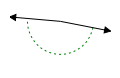### Explanation

Step 1:

Given angle is more than 90 and less than 180

Step 2:

So, the given angle is an obtuse angle.

Q 2 - Classify each angle as acute, obtuse, right or straight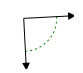### Explanation

Step 1:

Given angle is equal to 90.

Step 2:

So, the given angle is a right angle.

Q 3 - Classify each angle as acute, obtuse, right or straight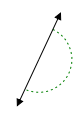### Explanation

Step 1:

Given angle is equal to 180.

Step 2:

So, the given angle is a straight angle

Q 4 - Classify each angle as acute, obtuse, right or straight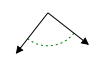### Explanation

Step 1:

Given angle is equal to 90.

Step 2:

So, the given angle is a right angle.

Q 5 - Classify each angle as acute, obtuse, right or straight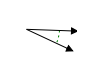### Explanation

Step 1:

Given angle is less than 90.

Step 2:

So, the given angle is an acute angle.

Q 6 - Classify each angle as acute, obtuse, right or straight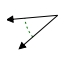### Explanation

Step 1:

Given angle is less than 90.

Step 2:

So, the given angle is an acute angle.

Q 7 - Classify each angle as acute, obtuse, right or straight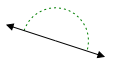### Explanation

Step 1:

Given angle is equal to 180.

Step 2:

So, the given angle is a straight angle

Q 8 - Classify each angle as acute, obtuse, right or straight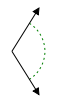### Explanation

Step 1:

Given angle is more than 90 and less than 180

Step 2:

So, the given angle is an obtuse angle

Q 9 - Classify each angle as acute, obtuse, right or straight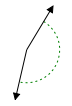### Explanation

Step 1:

Given angle is more than 90 and less than 180

Step 2:

So, the given angle is an obtuse angle

Q 10 - Classify each angle as acute, obtuse, right or straight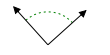### Explanation

Step 1:

Given angle is equal to 90.

Step 2:

So, the given angle is a right angle.

acute_obtuse_and_right_angles.htm